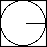# Mental Mat Quiz - non traditional algorithm

Mathematics is not about numbers, equations, computations, or algorithms: it is about understanding.

William Paul Thurston

Name _________________________________________

Directions: The idea is to work all problems in your head withour using a traditional algorithm. Write answers only. Take only ten minutes to complete it.

1. 48 + 39 = _______
2. 113 – 98 = _______
3. 14 x 5 x 7  = _______
4. 4 x 249 = _______
5. 6(37 + 63) + 18 = _______
6. .25 x 9 = _______
7. 1/2 + 1/3 = _______
8. 152 – 75 = _______
9. 85/39 is closest to what integer? _______
10. 12.03 + .4 + 2.36 = _______
11. If you buy items priced (tax included) at \$1.99, \$2.99 and \$3.98, your change from a \$10 bill would be? _______
12. 90% of 160 = _______
13. To the nearest dollar, the sale price of a dress listed at \$49.35 and sold at 25% off is _______.
14. The sum of the first ten odd positive integers (1 + 3 + 5 + 7 + 9 … 17 + 19) is _______.
15. The area of a square region of pe
16. rimeter of 20 is _______.
17. The ratio of the area of a circular region of radius one to that of the circumscribed square region is closest to .5,    .6,    .7 ,    .8,    .9

(Circular region with area of one and circumscribed square region)1. The average (arithmetic mean) of 89, 94, 85, 90 and 97 is _______.
2. If 4/6  = 16/x, then x = _______.
3. If 2x + 3 = 25, then x = _______.
4. 75 is closest to which integer? _______.
5. To the nearest dollar, a 15% tip on a restaurant bill of \$79.87 is _______.